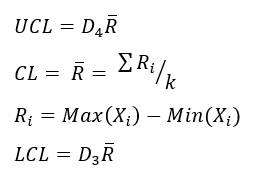# X Median R Chart Formulas

## QI Macros can calculate them for you!

#### Create an XmedianR Chart using QI Macros

2. Select XmedianR on QI Macros menu.
3. QI Macros will do the math and draw the graph for you.

Go Deeper:

The XMedianR Chart works just like the XbarR chart except that it uses the median instead of the average as a measure of central tendency.

### XMedianR Chart formulas for the Range and Median charts.

#### Range Chart Formula#### X Median Chart Formulan = number of samples in a subgroup

k = number of subgroups used to determine the average median and range

Conforms with AIAG SPC 2nd Edition

Process: Calculate, plot, and evaluate the range chart first. If it is "out of control," so is the process. If the range chart looks okay, then calculate, plot, and evaluate the X Chart.

Note: There are no known calculations for constants in an XMedianR Chart where n > 100

The XMedianR Chart is just one of the tools included in QI Macros SPC Software for Excel.

### Why Choose QI Macros Over Other Control Chart Software?### Fast and Easy to Use

• Works right in Excel
• Create charts in seconds
• Easy to customize & share charts
• Free Training Anytime### Proven and Trusted

• More than 100,000 users
• In More than 80 countries
• Five Star CNET Rating - Virus free### Affordable

• Only \$349 USD
Quantity Discounts Available
• No annual subscription fees
• Free technical support